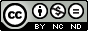Topological invariants of line arrangements

Guerville-Ballé, Benoît
Artal-Bartolo, Enrique (dir.) ; Florens, Vincent (dir.) ; Vallés, Jean (dir.)

Abstract: This thesis is the intersection point between the two facets of the study of line arrangements: combinatorics and topology. In the first part, we study the inclusion of the boundary manifold in the complement of an arrangement. We generalize the results of E. Hironaka to the case of any complex line arrangement. To get around the problems due to the case of non complexified real arrangement, we study the braided wiring diagram. We develop a Sage program to compute it from the equation of the complex line arrangement. This diagram allows to give two explicit descriptions of the map induced by the inclusion on the fundamental groups. From theses descriptions, we obtain two new presentations of the fundamental group of the complement. One of them is a generalization of the R. Randell Theorem to any complex line arrangement. In the next step of this work, we study the map induced by the inclusion on the first homology group. Then we obtain two simple descriptions of this map. Inspired by ideas of J.I. Cogolludo, we give a canonical description of the homology of the boundary manifold as the product of the 1-homology with the 2-cohomology of the complement. Finally, we obtain an isomorphism between the 2-cohomology of the complement with the 1-homology of the incidence graph of the arrangement. In the second part, we are interested by the study of character on the group of the complement. We start from the results of E. Artal on the computation of the depth of a character. This depth can be decomposed into a projective term and a quasi-projective term, vanishing for characters that ramify along all the lines. An algorithm to compute the projective part is given by A. Libgober. E. Artal focuses on the quasi-projective part and gives a method to compute it from the image by the character of certain cycles of the complement. We use our results on the inclusion map of the boundary manifold to determine these cycles explicitly. Combined with the work of E. Artal we obtain an algorithm to compute the quasi-projective depth of any character. From the study of this algorithm, we obtain a strong combinatorial condition on characters to admit a quasi-projective depth potentially not determined by the combinatorics. With this property, we define the inner-cyclic characters. From their study, we observe a strong condition on the combinatorics of an arrangement to have only characters with null quasi-projective depth. Related to this, in order to reduce the number of computations, we introduce the notion of prime combinatorics. If a combinatorics is not prime, then the characteristics varieties of its realizations are completely determined by realization of a prime combinatorics with less line. In parallel, we observe that the composition of the map induced by the inclusion with specific characters provide topological invariants of the blow-up of arrangements. We show that the invariant captures more than combinatorial information. Thereby, we detect two new examples of nc-Zariski pairs.

Pal. clave: topología ; geometría ; geometría algebraica

Knowledge area: Geometría y topología

Department: Instituto Universitario de Investigación de Matemáticas y Aplicaciones (IUMA)

Nota: Tesis-Univ. Zaragoza, Instituto Universitario de Investigación de Matemáticas y Aplicaciones (IUMA), 2013